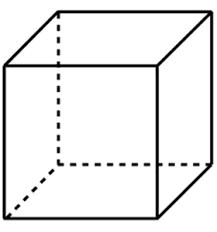# Surface and volume od cuboid

Content area of the square base of cuboid is Sp = 36 cm2 and its height 80 mm. Determine its surface area and volume.

S =  264 cm2
V =  288 cm3

### Step-by-step explanation:Did you find an error or inaccuracy? Feel free to write us. Thank you!Tips to related online calculators
Do you want to convert length units?
Do you know the volume and unit volume, and want to convert volume units?

#### You need to know the following knowledge to solve this word math problem:

We encourage you to watch this tutorial video on this math problem:

## Related math problems and questions:

• The regularThe regular triangular prism has a base in the shape of an isosceles triangle with a base of 86 mm and 6.4 cm arms, the height of the prism is 24 cm. Calculate its volume.
• Triangular prismThe base of the perpendicular triangular prism is a right triangle with a leg length of 5 cm. The content area of the largest sidewall of its surface is 130 cm², and the height of the body is 10 cm. Calculate its volume.
• Tetrahedral prism - rhomboid baseCalculate the area and volume tetrahedral prism that has base rhomboid shape and its dimensions are: a = 12 cm, b = 70 mm, v_a = 6 cm, v_h = 1 dm.
• Water tankWhat is the height of the cuboid-shaped tank with the bottom dimensions of 80 cm and 50 cm if the 480 liters of water reaches 10 cm below the top?
• Four prismsQuestion No. 1: The prism has the dimensions a = 2.5 cm, b = 100 mm, c = 12 cm. What is its volume? a) 3000 cm2 b) 300 cm2 c) 3000 cm3 d) 300 cm3 Question No.2: The prism base is a rhombus with a side length of 30 cm and a height of 27 cm. The height of t
• PrismThe base of the prism is a rhombus with a side 30 cm and a height 27 cm long. The height of the prism is 180% longer than the side length of the rhombus. Calculate the volume of the prism.
• Tetrahedral prismCalculate surface and volume tetrahedral prism, which has a rhomboid-shaped base, and its dimensions are: a = 12 cm, b = 7 cm, ha = 6 cm and prism height h = 10 cm.
• Triangular prismCalculate the volume and surface of the triangular prism ABCDEF with base of a isosceles triangle. Base's height is 16 cm, leg 10 cm, base height vc = 6 cm. The prism height is 9 cm.
• Prism - boxThe base of prism is a rectangle with a side of 7.5 cm and 12.5 cm diagonal. The volume of the prism is V = 0.9 dm3. Calculate the surface of the prism.
• The surfaceThe cuboid's surface area is 1714 cm2, the edges of the base are 25 cm and 14 cm long. Find the area of the surface.
• Square prismCalculate the volume of a foursided prism 2 dm high, the base is a trapezoid with bases 12 cm, 6 cm, height of 4 cm and 5 cm long arms.
• Triangular prismCalculate a triangular prism if it has a rectangular triangle base with a = 4cm and hypotenuse c = 50mm and height of the prism is 0.12 dm.
• Surface areaCalculate the surface area of a four-sides 2-m high prism which base is a rectangle with sides 17 cm and 1.3 dm
• Trapezoidal prismCalculate the surface of the quadrilateral prism ABCDA'B'C'D 'with the trapezoidal base ABCD. The height of the prism is 12 cm; ABCD trapezoidal data: AB base length is 8 cm, CD base length is 3 cm, BC arm length is 4 cm, and AC diagonal length is 7 cm. L
• Prism 4 sidesFind the surface area and volume four-sided prism high 10cm if its base is a rectangle measuring 8 cm and 1.2dm
• PrismCalculate the surface area and volume of a prism with a body height h = 10 cm, and its base has the shape of a rhomboid with sides a = 5.8 cm, b = 3 cm, and the distance of its two longer sides is w = 2.4 cm.
• Prism 4 sidesThe prism has a square base with a side length of 3 cm. The diagonal of the sidewall of the prism/BG/is 5 cm. Calculate the surface of this prism in cm square and the volume in liters